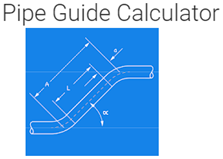# 7 – Best pipe calculator apps for android and their features

1. Pipe diameter calculator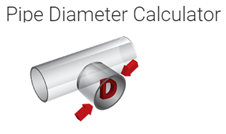This is a calculator app that can be used to calculate the diameter of the pipe, by using this calculator we can calculate the internal diameter of the pipe and this calculation is done by using the volume and mass flow rate and also the desired stream velocity through the pipe

2. Pipe volume calculator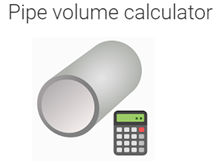By using this app we can calculate the volume of any pipe, in order to calculate the volume of the pipe we need to get the diameter and length of the pipe and by this, we could get the pipe volume. This app will be very helpful for students and to people who do any manual job where the pipe is used.

3. Water head-loss calculatorBy using this app we can calculate the pressure drop of water in a circular pipe which is caused by friction. In order to do the calculation, it requires certain input parameters and they are flow, pipe diameter, length of the pipe. This app would be very useful for piping engineers.

4. Pipeflex calculator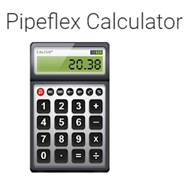This app will be very useful for piping engineers and students, this app has many piping equations and formulas. This app has a memory mode and by this, we can store data as much as we want. It can calculate the volume of welding material that is required to weld the pipe. It can also calculate the flow rate, velocity, and pipe diameter. It can calculate the complex roll offset and simple roll offset. This app also has a converter that can be used to convert the pressure, temperature, weight, etc.

5. Piping calculators freeThis app will be very useful for piping engineers to do calculations for chemical, petrochemical, and other industrial plants. By using this app we can do the calculations like pipe spacing, pressure calculation, pipe wall thickness calculations, elbow center calculations, insulation thickness calculations, and lot more.

6. Calculator pipe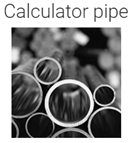By using this app we can calculate the mass of round pipes. It also has a certain formula which can be used to calculate the mass of rectangular and square pipes. Calculations are done by using certain details such as the density of the material, external diameter, etc.